Single Phase Fully Controlled Converters

# Single Phase Fully Controlled Converters - Notes | Study Power Electronics - Electrical Engineering (EE)

 1 Crore+ students have signed up on EduRev. Have you?

Operation and Analysis of single phase fully controlled converter.

Instructional Objectives

On completion the student will be able to

• Differentiate between the constructional and operation features of uncontrolled and controlled converters
• Draw the waveforms and calculate their average and RMS values of different variables associated with a single phase fully controlled half wave converter.
• Explain the operating principle of a single phase fully controlled bridge converter.
• Identify the mode of operation of the converter (continuous or discontinuous) for a given load parameters and firing angle.
• Analyze the converter operation in both continuous and discontinuous conduction mode and there by find out the average and RMS values of input/output, voltage/currents.
• Explain the operation of the converter in the inverter mode.

Introduction

Single phase uncontrolled rectifiers are extensively used in a number of power electronic based converters. In most cases they are used to provide an intermediate unregulated dc voltage source which is further processed to obtain a regulated dc or ac output. They have, in general, been proved to be efficient and robust power stages. However, they suffer from a few disadvantages. The main among them is their inability to control the output dc voltage / current magnitude when the input ac voltage and load parameters remain fixed. They are also unidirectional in the sense that they allow electrical power to flow from the ac side to the dc side only. These two disadvantages are the direct consequences of using power diodes in these converters which can block voltage only in one direction. As will be shown in this module, these two disadvantages are overcome if the diodes are replaced by thyristors, the resulting converters are called fully controlled converters.
Thyristors are semicontrolled devices which can be turned ON by applying a current pulse at its gate terminal at a desired instance. However, they cannot be turned off from the gate terminals. Therefore, the fully controlled converter continues to exhibit load dependent output voltage / current waveforms as in the case of their uncontrolled counterpart. However, since the thyristor can block forward voltage, the output voltage / current magnitude can be controlled by controlling the turn on instants of the thyristors. Working principle of thyristors based single phase fully controlled converters will be explained first in the case of a single thyristor halfwave rectifier circuit supplying an R or R-L load. However, such converters are rarely used in practice.

Full bridge is the most popular configuration used with single phase fully controlled rectifiers. Analysis and performance of this rectifier supplying an R-L-E load (which may represent a dc motor) will be studied in detail in this lesson.

Single phase fully controlled halfwave rectifier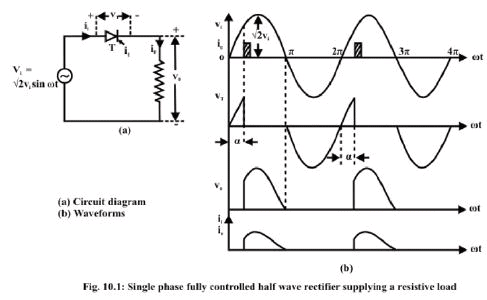Fig.10. 1(a) shows the circuit diagram of a single phase fully controlled halfwave rectifier supplying a purely resistive load. At ωt = 0 when the input supply voltage becomes positive the thyristor T becomes forward biased. However, unlike a diode, it does not turn ON till a gate pulse is applied at ωt = α. During the period 0 < ωt ≤ α, the thyristor blocks the supply voltage and the load voltage remains zero as shown in fig 10.1(b). Consequently, no load current flows during this interval. As soon as a gate pulse is applied to the thyristor at ωt = α it turns ON. The voltage across the thyristor collapses to almost zero and the full supply voltage appears across the load. From this point onwards the load voltage follows the supply voltage. The load being purely resistive the load current io is proportional to the load voltage. At ωt = π as the supply
voltage passes through the negative going zero crossing the load voltage and hence the load current becomes zero and tries to reverse direction. In the process the thyristor undergoes reverse recovery and starts blocking the negative supply voltage. Therefore, the load voltage and the load current remains clamped at zero till the thyristor is fired again at ωt = 2π + α . The same process repeats there after.

Fig 10.2 (a) and (b) shows the circuit diagram and the waveforms of a single phase fully controlled halfwave rectifier supplying a resistive inductive load. Although this circuit is hardly used in practice its analysis does provide useful insight into the operation of fully controlled rectifiers which will help to appreciate the operation of single phase bridge converters to be discussed later.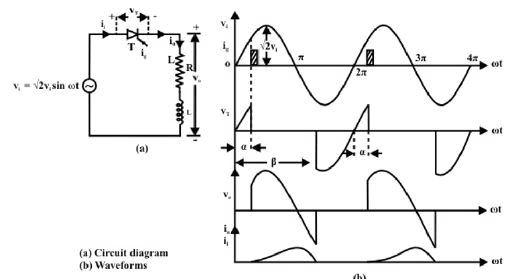Single phase fully controlled bridge converter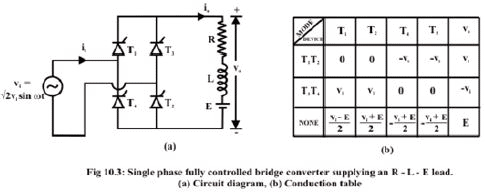Fig 10.3 (a) shows the circuit diagram of a single phase fully controlled bridge converter. It is one of the most popular converter circuits and is widely used in the speed control of separately excited dc machines. Indeed, the R-L-E load shown in this figure may represent the electrical equivalent circuit of a separately excited dc motor.

The single phase fully controlled bridge converter is obtained by replacing all the diode of the corresponding uncontrolled converter by thyristors. Thyristors T1 and Tare fired together while Tand T4 are fired 180º after Tand T2. From the circuit diagram of Fig 10.3(a) it is clear that for any load current to flow at least one thyristor from the top group (T1, T3) and one thyristor from the bottom group (T2, T4) must conduct. It can also be argued that neither T1T3 nor T2T4 can conduct simultaneously. For example whenever T3 and T4 are in the forward blocking state and a gate pulse is applied to them, they turn ON and at the same time a negative voltage is applied across T1 and T2 commutating them immediately. Similar argument holds for T1 and T2.

For the same reason T1T4 or T2T3 can not conduct simultaneously. Therefore, the only possible conduction modes when the current i0 can flow are T1T2 and T3T4. Of coarse it is possible that at a given moment none of the thyristors conduct. This situation will typically occur when the load current becomes zero in between the firings of T1T2 and T3T4. Once the load current becomes zero all thyristors remain off. In this mode the load current remains zero.

Consequently the converter is said to be operating in the discontinuous conduction mode.

Fig 10.3(b) shows the voltage across different devices and the dc output voltage during each of these conduction modes. It is to be noted that whenever T1 and Tconducts, the voltage across T3 and T4 becomes -vi. Therefore T3 and T4 can be fired only when vi is negative i.e, over the negative half cycle of the input supply voltage. Similarly T1 and T2 can be fired only over the positive half cycle of the input supply. The voltage across the devices when none of the thyristors conduct depends on the off state impedance of each device. The values listed in Fig 10.3 (b) assume identical devices.
Under normal operating condition of the converter the load current may or may not remain zero over some interval of the input voltage cycle. If i0 is always greater than zero then the converter is said to be operating in the continuous conduction mode. In this mode of operation of the converter T1T2 and T3T4 conducts for alternate half cycle of the input supply.
However, in the discontinuous conduction mode none of the thyristors conduct over some portion of the input cycle. The load current remains zero during that period.

Operation in the continuous conduction mode

As has been explained earlier in the continuous conduction mode of operation i0 never becomes zero, therefore, either T1T2 or T3T4 conducts. Fig 10.4 shows the waveforms of different variables in the steady state. The firing angle of the converter is . The angle is given by It is assumed that at ωt = 0 - T3T4 was conducting. As T1T2 are fired at ωt =  α they turn on commutating T3T4 immediately. T3T4 are again fired at ωt = π + α . Till this point T1T2
conducts. The period of conduction of different thyristors are pictorially depicted in the second waveform (also called the conduction diagram) of Fig 10.4.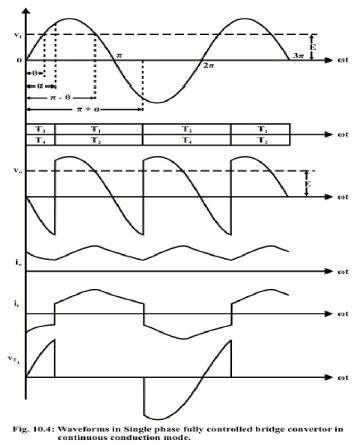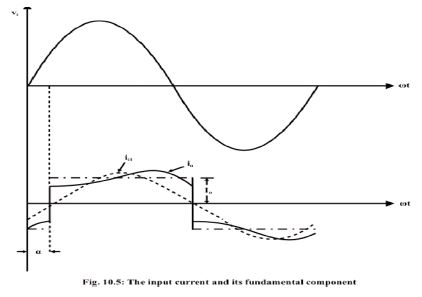It will be of interest to find out a Fourier series expression of ii. However, using actual expression for ii will lead to exceedingly complex calculation. Significant simplification can be made by replacing i0 with its average value I0. This will be justified provided the load is highly inductive and the ripple on i0 is negligible compared to I0. Under this assumption the idealized waveform of ii becomes a square wave with transitions at ωt = α and ωt = π + α as shown in Fig 10.5. ii1 is the fundantal component of this idealized ii. Evidently the input current displacement factor defined as the cosine of the angle between input voltage (vi) and the fundamental component of input current (ii1) waveforms is cos (lagging).

Therefore, the rectifier appears as a lagging power factor load to the input ac system. Larger the '' poorer is the power factor.
The input current ii also contain significant amount of harmonic current (3rd, 5th, etc) and therefore appears as a harmonic source to the utility. Exact composition of the harmonic currents can be obtained by Fourier series analysis of ii and is left as an exercise.

Fill in the blank(s) with the appropriate word(s).

i) A single phase fully controlled bridge converter can operate either in the _________ or ________ conduction mode.

ii) In the continuous conduction mode at least _________ thyristors conduct at all times.

iii) In the continuous conduction mode the output voltage waveform does not depend on the ________ parameters.

iv) The minimum frequency of the output voltage harmonic in a single phase fully controlled bridge converter is _________ the input supply frequency.

v) The input displacement factor of a single phase fully controlled bridge converter in the continuous conduction mode is equal to the cosine of the ________ angle.

Answer: (i) continuous, discontinuous; (ii) two; (iii) load; (iv) twice; (v) firing.

Operation in the discontinuous conduction mode

So far we have assumed that the converter operates in continuous conduction mode without paying attention to the load condition required for it. In figure 10.4 the voltage across the R and L component of the load is negative in the region π - θ ≤ ωt ≤ π + α . Therefore i0 continues to decrease till a new pair of thyristor is fired at ωt = π + α . Now if the value of R, L and E are such that i0 becomes zero before ωt = π + α the conduction becomes discontinuous. Obviously then, at the boundary between continuous and discontinuous conduction the minimum value of iwhich occurs at ωt = α = and  ωt = π + α will be zero. Putting this condition in (10.26) we obtain the condition for continuous conduction as.
Fig 10.6 shows waveforms of different variables on the boundary between continuous and discontinuous conduction modes and in the discontinuous conduction mode. It should be stressed that on the boundary between continuous and discontinuous conduction modes the load current is still continuous. Therefore, all the analysis of continuous conduction mode applies to this case as well. However in the discontinuous conduction mode i0 remains zero for certain interval.

During this interval none of the thyristors conduct. These intervals are shown by hatched lines in the conduction diagram of Fig 10.6(b). In this conduction mode istarts rising from zero as T1T2 are fired at ωt = α . The load current continues to increase till ωt = π - θ. After this, the output voltage v0 falls below the emf E and i0 decreases till ωt = β  when it becomes zero. Since the thyristors cannot conduct current in the reverse direction i0 remains at zero till ωt = π + α when T3 and T4 are fired. During the period β ≤ ωt ≤ π + α none of the thyristors conduct. During this period v0 attains the value E.

Performance of the rectifier such as VOAV, VORMS, IOAV, IORMS etc can be found in terms of α, β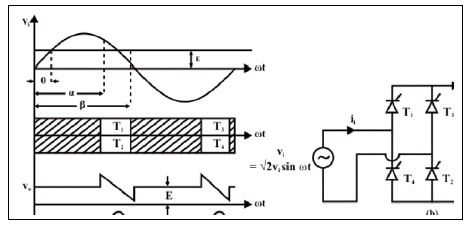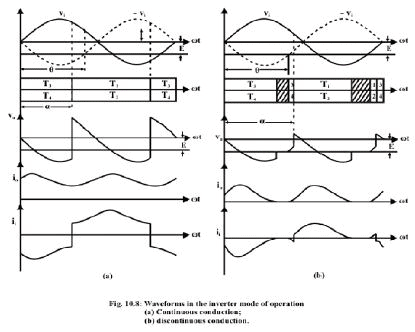The document Single Phase Fully Controlled Converters - Notes | Study Power Electronics - Electrical Engineering (EE) is a part of the Electrical Engineering (EE) Course Power Electronics.
All you need of Electrical Engineering (EE) at this link: Electrical Engineering (EE)

## Power Electronics

5 videos|39 docs|63 tests
 Use Code STAYHOME200 and get INR 200 additional OFF

## Power Electronics

5 videos|39 docs|63 tests

Track your progress, build streaks, highlight & save important lessons and more!

,

,

,

,

,

,

,

,

,

,

,

,

,

,

,

,

,

,

,

,

,

;